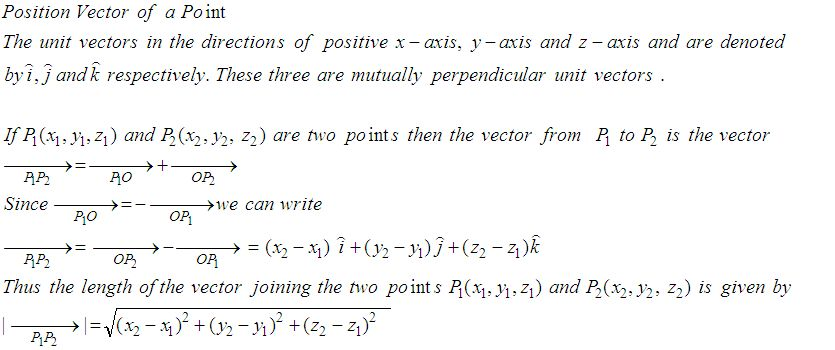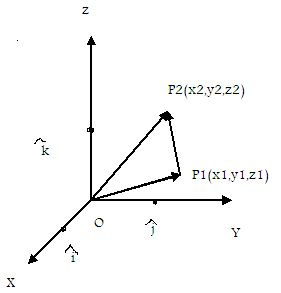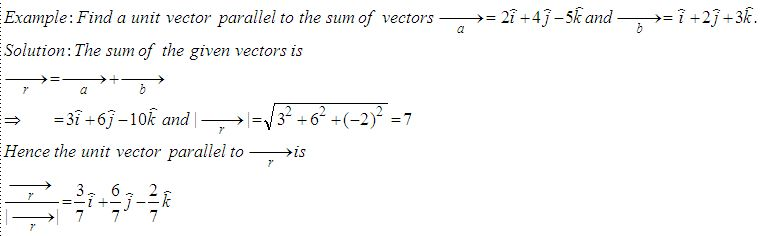Name: ___________________Date:___________________

 Email us to get an instant 20% discount on highly effective K-12 Math & English kwizNET Programs!

### High School Mathematics - 25.9 Position Vector of a PointDirections: Answer the following.
 Q 1: Find the unit vector in the direction of P(1,2,3) towards Q(4,5,6).6i+5j+2k(i+j+k)/Ö38j-2k Q 2: If P = 2,4,7 and P2 = (-4, -1, 5) then find the magnitude of vector P1P2.3i+6jÖ656i+5j Question 3: This question is available to subscribers only! Question 4: This question is available to subscribers only!